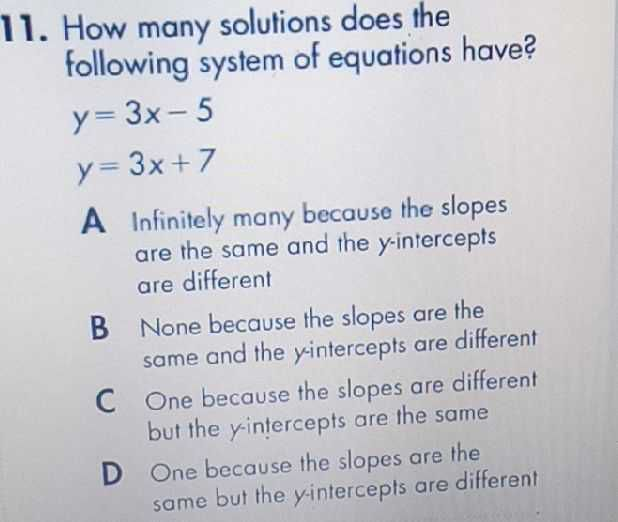### ¿Todavía tienes preguntas de matemáticas?

Pregunte a nuestros tutores expertos
Algebra
Pregunta11. How many solutions does the following system of equations have?

$$y = 3 x - 5$$

$$y = 3 x + 7$$

A Infinitely many because the slopes are the same and the $$y$$ -intercepts are different

B None because the slopes are the same and the y-intercepts are different

C One because the slopes are different but the $$y$$ -intercepts are the same

D One because the slopes are the same but the y-intercepts are different